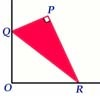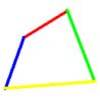#### You may also like### Set Square

A triangle PQR, right angled at P, slides on a horizontal floor with Q and R in contact with perpendicular walls. What is the locus of P?### Biggest Bendy

Four rods are hinged at their ends to form a quadrilateral. How can you maximise its area?### Strange Rectangle 2

Find the exact values of some trig. ratios from this rectangle in which a cyclic quadrilateral cuts off four right angled triangles.

# Strange Rectangle

##### Age 16 to 18 Challenge Level:

To crack this Tough Nut look for pairs of similar triangles and angles adding up to $90$ degrees and this leads to $PQRS$ being a cyclic quadrilateral with $SQ$ as diameter. You need to know that opposite angles of a cyclic quadrilateral add up to $180$ degrees and the angle at the centre of a circle is twice the angle at the circumference subtended by the same arc. Call the centre of the circle $O$ the use the converse of Pythagoras' Theorem to find the angle $POR$ . The rest follows.